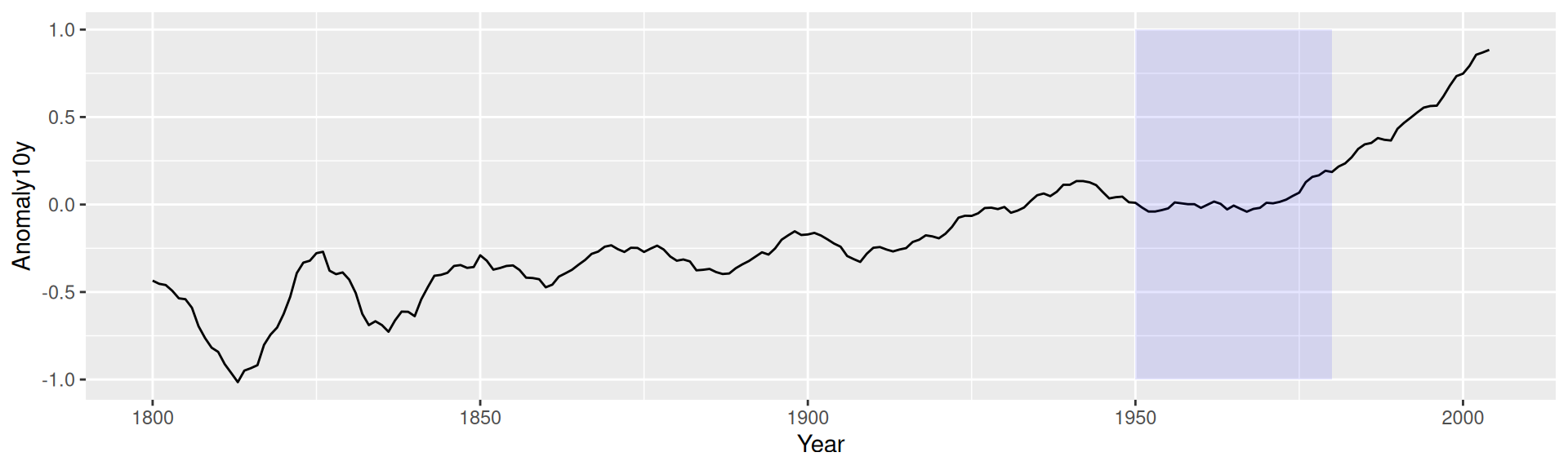### 7.5.2 Solution

Use `annotate("rect")` (Figure 7.12):

``````library(gcookbook) # Load gcookbook for the climate data set

p <- ggplot(filter(climate, Source == "Berkeley"), aes(x = Year, y = Anomaly10y)) +
geom_line()

p +
annotate("rect", xmin = 1950, xmax = 1980, ymin = -1, ymax = 1,
alpha = .1,fill = "blue")``````Any geom can be used with `annotate()`, as long as you pass in the proper parameters. In this case, `geom_rect()` requires min and max values for x and y.